Lesson Progress
0% Complete

## 1.1 POSITIVE AND NEGATIVE INTEGERS:

When two positive integers are added we get a positive integer.

eg: +7  + 5  = + 12

When two negative  integers are added we get a negative integer

eg: −8 + −5 = −13

When one positive integer and one negative integer are added we get a negative or a positive integer.

Case I :  When the positive integer is bigger

+ 36+( −21) = 15

Case II : When the positive integer is smaller

(−36) + (+21) = −15

## Exercise 1.1

1. Following number line shows the temperature in degree Celsius (Co) at different places on a particular day.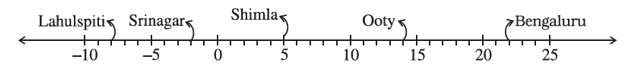(a) Observe this number line and write the temperature of the places marked on it.

Solution:  By observing the number line, we can find the temperature of the cities as follows,

Temperature at  Lahulspiti  is   – 8oC

Temperature at  Srinagar  is   –2oC

Temperature at  Shimla is   5oC

Temperature at  Ooty  is   14oC

Temperature at  Bengaluru  is   22oC

(b) What is the temperature difference between the hottest and the coldest places among the above?

Solution:–   From the number line we observe that,

The temperature at the hottest place  Bengaluru is  22oC

The temperature at the coldest place Lahulspiti is  – 8oC

Temperature difference between hottest and coldest place is = 22oC –  (– 8oC)

=  22o C + 8o C       =  30o C

Hence, the temperature difference between the hottest and the coldest place is 30o C.

(c) What is the temperature difference between Lahulspiti and Srinagar?

Solution:– From the given number line,

The temperature at the Lahulspiti is  –8o C

The temperature at the Srinagar is  –2o C

∴ The temperature difference between Lahulspiti and Srinagar is = –2o C – (−8o C)

= – 2° C + 8o C    = 6o C

∴ The temperature difference between Lahulspiti and Srinagar is 6o C

(d) Can we say temperature of Srinagar and Shimla taken together is less than the

temperature at Shimla? Is it also less than the temperature at Srinagar?

Solution:–  From the given number line,

The temperature at Srinagar = –2o C

The temperature at Shimla = 5o C

The temperature of Srinagar and Shimla taken together is = – 2o C + 5o C    = 3o C

∴ 5o C  >  3o C

So, the temperature of Srinagar and Shimla taken together is less than the temperature at Shimla.

Then,    3o >  − 2o

No, the temperature of Srinagar and Shimla taken together is not less than the temperature of Srinagar.

2.In a quiz, positive marks are given for correct answers and negative marks are given   for incorrect answers. If Jack’s scores in five successive rounds were 25, 5,   – 10, 15 and 10, what was his total at the end?

Solution:–   Jack’s score in five successive rounds are 25, − 5,  −10,  15 and  10

The total score of Jack at the end will be = 25 + (5) + (10) + 15 + 10

=  25 – 5 – 10 + 15 + 10    =  50 – 15     =  35

∴ Jack’s total score at the end is 35.

3.At Srinagar temperature was – 5°C on Monday and then it dropped by 2°C on Tuesday. What was the temperature of Srinagar on Tuesday? On Wednesday, it rose by 4°C. What was the temperature on this day?

Solution: Temperature on Monday at Srinagar = – 5o C

Temperature on Tuesday at Srinagar is dropped by 2o = Temperature on Monday – 2o C

= – 5o C – 2o C    = – 7o C

Temperature on Wednesday at Srinagar is rose by  4o = Temperature on Tuesday  + 4o C

= –  7o C + 4o C    = – 3o C

Thus, the temperature on Tuesday and Wednesday was –7o C and –3o C respectively.

4.A plane is flying at the height of 5000 m above the sea level. At a particular point, it is exactly above a submarine floating 1200 m below the sea level. What is the vertical distance between them?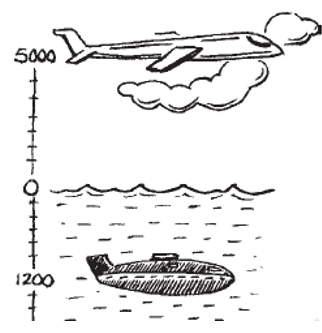Solution:–   Plane is flying at the height = 5000 m

Depth of Submarine = –1200 m

The vertical distance between plane and submarine = 5000 m – (– 1200) m

= 5000 m + 1200 m      = 6200 m

The vertical distance between plane and submarine = 6200 m

5. Mohan deposits ₹ 2,000 in his bank account and withdraws ₹ 1,642 from it, the next day. If withdrawal of amount from the account is represented by a negative integer, then how will you represent the amount deposited? Find the balance in Mohan’s account after the withdrawal.

Solution:–  Withdrawal of amount from the account is represented by a negative integer.

Then, deposit of amount to the account is represented by a positive integer

Total amount deposited in bank account by the Mohan =  +₹ 2000

Total amount withdrawn from the bank account by the Mohan = – ₹ 1642

Balance in Mohan’s account after the withdrawal = amount deposited + amount withdrawn

= ₹ 2000 + (–₹ 1642)    = ₹ 2000 – ₹ 1642     = ₹ 358

Hence, the balance in Mohan’s account after the withdrawal is ₹ 358

6. Rita goes 20 km towards east from a point A to the point B. From B, she moves 30 km towards west along the same road. If the distance towards east is represented by a positive integer then, how will you represent the distance travelled towards west? By which integer will you represent her final position from A?Solution:–    It is given that

A positive integer represents the distance towards the east.

Then, distance travelled towards the west will be represented by a negative integer.

Rita travels a distance in east direction = 20 km

Rita travels a distance in west direction = – 30 km

∴ Distance travelled from A = 20 + (– 30)

= 20 – 30   = –10 km

Hence, we will represent the distance travelled by Rita from point A by a negative integer,– 10 km

7.In a magic square each row, column and diagonal have the same sum. Check which of the following is a magic square.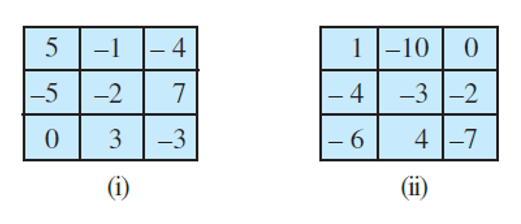Solution:–  First we consider the square (i)

By adding the numbers in each row we get,

= 5 + (– 1) + (– 4) = 5 – 1 – 4 = 5 – 5 = 0

= –5 + (–2) + 7 = – 5 – 2 + 7 = –7 + 7 = 0

= 0 + 3 + (–3) = 3 – 3 = 0

By adding the numbers in each column we get,

= 5 + (– 5) + 0 = 5 – 5 = 0

= (–1) + (–2) + 3 = –1 – 2 + 3 = –3 + 3 = 0

= –4 + 7 + (–3) = – 4 + 7 – 3 = –7 + 7 = 0

By adding the numbers in the diagonals we get,

= 5 + (–2) + (–3) = 5 – 2 – 3 = 5 – 5 = 0

= –4 + (–2) + 0 = – 4 – 2 = –6

Because sum of one diagonal is not equal to zero,

So, (i) is not a magic square

Now, we consider the square (ii)

By adding the numbers in each rows we get,

= 1 + (–10) + 0 = 1 – 10 + 0 = –9

= (–4) + (–3) + (–2) = –4 – 3 – 2 = –9

= (–6) + 4 + (–7) = –6 + 4 – 7 = –13 + 4 = –9

By adding the numbers in each columns we get,

= 1 + (– 4) + (–6) = 1 – 4 – 6 = 1 – 10 = –9

= (–10) + (–3) + 4 = –10 – 3 + 4 = –13 + 4 =−9

= 0 + (–2) + (–7) = 0 – 2 – 7 = –9

By adding the numbers in diagonals we get,

= 1 + (–3) + (–7) = 1 – 3 – 7 = 1 – 10 = –9

= 0 + (–3) + (–6) = 0 – 3 – 6 = –9

This (ii) square is a magic square, because sum of each row, each column and diagonal is equal.

8.Verify a – (– b) = a + b for the following values of a and b.

(i) a = 21, b = 18

Solution:–   From the question,  a = 21 and b = 18

To verify a – (– b) = a + b

Let us take Left Hand Side (LHS) = a – (– b)

= 21 – (– 18)   = 21 + 18   = 39

Now, Right Hand Side (RHS) = a + b

= 21 + 18  = 39

By comparing LHS and RHS  we get   LHS = RHS

∴  39 = 39

Hence, the value of a and b is verified.

(ii) a = 118, b = 125

Solution:–  From the question,

a = 118 and b = 125

To verify a – (– b) = a + b

Let us take Left Hand Side (LHS) = a – (– b)

= 118 – (– 125)   = 118 + 125  = 243

Now, Right Hand Side (RHS) = a + b

= 118 + 125    = 243

By comparing LHS and RHS, we get ,  LHS = RHS

∴  243 = 243

Hence, the value of a and b is verified.

(iii) a = 75, b = 84

Solution:–From the question,

a = 75 and b = 84

To verify  a – (– b) = a + b

Let us take Left Hand Side (LHS) = a – (– b)

= 75 – (– 84)   = 75 + 84   = 159

Now, Right Hand Side (RHS) = a + b

= 75 + 84  = 159

By comparing LHS and RHS,   we get,  LHS = RHS

∴ 159 = 159

Hence, the value of a and b is verified.

(iv) a = 28, b = 11

Solution:–  From the question,

a = 28 and b = 11

To verify a – (– b) = a + b

Let us take Left Hand Side (LHS) = a – (– b)

= 28 – (– 11)  = 28 + 11  = 39

Now, Right Hand Side (RHS) = a + b

= 28 + 11 = 39

By comparing LHS and RHS,   we get,  LHS = RHS

∴ 39 = 39

Hence, the value of a and b is verified.

9.Use the sign of >, < or = in the box to make the statements true.

(a) (–8) + (–4) [ ] (–8) – (–4)

Solution:–  Let us take Left Hand Side (LHS) = (–8) + (–4)

= –8 – 4   = –12

Now, Right Hand Side (RHS) = (–8) – (–4)

= –8 + 4  = –4

By comparing LHS and RHS,  we get,  LHS < RHS

So we get –12 < –4

∴ (–8) + (–4)  [<]  (–8) – (–4)

(b) (–3) + 7 – (19) [ ] 15 – 8 + (–9)

Solution:– Let us take Left Hand Side (LHS) = (–3) + 7 – 19

= – 3 + 7 – 19  = – 22 + 7  = – 15

Now, Right Hand Side (RHS) = 15 – 8 + (–9)

= 15 – 8 – 9  = 15 – 17  = –2

By comparing LHS and RHS, we get,   LHS < RHS

So we get  –15 < –2

∴ (–3) + 7 – (19)  [<]  15 – 8 + (–9)

(c) 23 – 41 + 11 [ ] 23 – 41 – 11

Solution:–  Let us take Left Hand Side (LHS) = 23 – 41 + 11

= 34 – 41  = – 7

Now, Right Hand Side (RHS) = 23 – 41 – 11

= 23 – 52  = – 29

By comparing LHS and RHS, we get,   LHS > RHS

So we get – 7 > –29

∴ 23 – 41 + 11 [>] 23 – 41 – 11

(d) 39 + (–24) – (15) [ ] 36 + (–52) – (– 36)

Solution:–Let us take Left Hand Side (LHS) = 39 + (–24) – 15

= 39 – 24 – 15  = 39 – 39  = 0

Now, Right Hand Side (RHS) = 36 + (–52) – (– 36)

= 36 – 52 + 36  = 72 – 52  = 20

By comparing LHS and RHS, we get,  LHS < RHS

So we get  0 < 20

∴ 39 + (–24) – (15) [<] 36 + (–52) – (– 36)

(e) – 231 + 79 + 51 [ ] –399 + 159 + 81

Solution:– Let us take Left Hand Side (LHS) = – 231 + 79 + 51

= – 231 + 130  = –101

Now, Right Hand Side (RHS) = – 399 + 159 + 81

= – 399 + 240  = – 159

By comparing LHS and RHS, we get,   LHS > RHS

So we get  –101 > –159

∴ – 231 + 79 + 51 [>] –399 + 159 + 81

10.A water tank has steps inside it. A monkey is sitting on the topmost step (i.e., the first step). The water level is at the ninth step.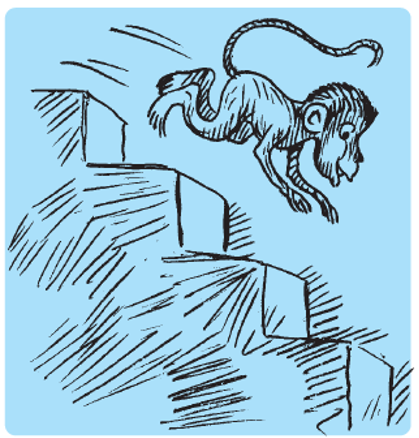(i) He jumps 3 steps down and then jumps back 2 steps up. In how many jumps will he reach the water level?

(ii) After drinking water, he wants to go back. For this, he jumps 4 steps up and then jumps back 2 steps down in every move. In how many jumps will he reach back the top step?

(iii) If the number of steps moved down is represented by negative integers and the number of steps moved up by positive integers, represent his moves in part (i) and (ii) by completing the following; (a) – 3 + 2 – … = – 8 (b) 4 – 2 + … = 8. In (a) the sum (– 8) represents going down by eight steps. So, what will the sum 8 in (b) represent?

Solution:– i) The monkey is sitting on the top (i.e., the first step)

The position of monkey after the

1st jump monkey will be at step = 1 + 3 = 4 steps

2nd jump monkey will be at step = 4 + (-2) = 4 – 2 = 2 steps

3rd jump monkey will be at step = 2 + 3 = 5 steps

4th jump monkey will be at step = 5 + (-2) = 5 – 2 = 3 steps

5th jump monkey will be at step = 3 + 3 = 6 steps

6th jump monkey will be at step = 6 + (-2) = 6 – 2 = 4 steps

7th jump monkey will be at step = 4 + 3 = 7 steps

8th jump monkey will be at step = 7 + (-2) = 7 – 2 = 5 steps

9th jump monkey will be at step = 5 + 3 = 8 steps

10th jump monkey will be at step = 8 + (-2) = 8 – 2 = 6 steps

11th jump monkey will be at step = 6 + 3 = 9 steps

∴ Monkey took 11 jumps (i.e., 9th step) to reach the water level

Thus, required number of jumps = 11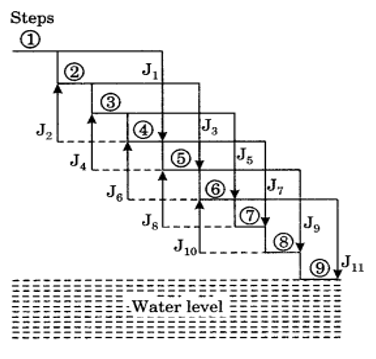ii) The monkey’s position is at the water level (the ninth step.)

The position of monkey after the

1st jump  J1 is at  5th step ↑
2nd jump  J2 is at  7th step ↓
3rd jump  J3 is at  3rd step ↑
4th jump  J4 is a t 5th step ↓
5th jump  J5 is at  1st step ↑
Hence, the required number of jumps = 5

1st jump  J1 is at  5th step ↑
2nd jump  J2 is at  7th step ↓
3rd jump  J3 is at  3rd step ↑
4th jump  J4 is at  5th step ↓
5th jump  J5 is at  1st step ↑
Thus, the required number of jumps = 5.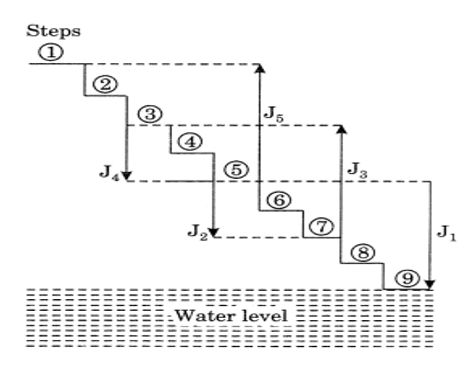iii) Since, the number of steps moved by the monkey towards the top is taken as positive and the number of steps moved towards the water level is taken as negative.

In case (i), we have:

 Jumps J1 J2 J3 J4 J5 J6 J7 J8 J9 J10 J11 Number of steps −      3 + 2 3 + 2 -3 + 2 3 + 2 3 + 2 3

Therefore (i) Total number of steps
=  3 + 2 – 3 + 2 – 3 + 2 – 3 + 2 – 3 + 2 – 3
=  8 which represents the monkey goes down by 8 steps.

In case (ii), we have:

 Jumps J1 J2 J3 J4 J5 Number of steps + 4 2 + 4 2 +4

Therefore (ii) Total number of steps.
= + 4 – 2 + 4 – 2 + 4 = 8
Here, the monkey is going up by 8 steps. Therefore in (b), the sum (+8) will represent going up by 8 steps.

Try these (page 8)

1.Write a pair of integers whose sum gives

a) A negative integer

b) Zero

c) An integer smaller than both the integers.

d) An integer  greater than both the integers.

e) An integer greater than both the integers.

Solution:–  a) (−16) and 9

Sum: (−16) + 9 = −7 which is negative integer.

b) −18 and 18

Sum: =  −18 + 18 = 0 which is zero

c) (−6) and (−8)

Sum: (−6) + (−8) = − 14

which is smaller than (−6) and (−8)

d) 4 and 7

Sum: 4 + 7 = 11

which is greater than 4 and 7

e) 19 and 21

Sum: 19 + 21 = 40

40 is greater than 19 and 21

1. Write a pair of integers whose difference gives a) A negative integer  b) Zero   c) An integer smaller than both the integers.  d) An integer greater than both the integers.  e) An integer greater than both the integers.

Solution:–  a) 3 and 7

Difference 3−7 = −4 which is a negative integer.

b) −9 and −9

Difference: −9 − (−9) = −9 + 9 = 0  which is zero

c) 5 and 9

Difference:   5−9 = −4 which is smaller than 5 and −9

d) 16 and −5

Difference: 16 −(− 5 )= 16 + 5 = 21 which is greater than 16 and −5

e) 15 and 6

Difference: 15  = 15 + 6 = 21

21 is greater than 15 as well as 6 .